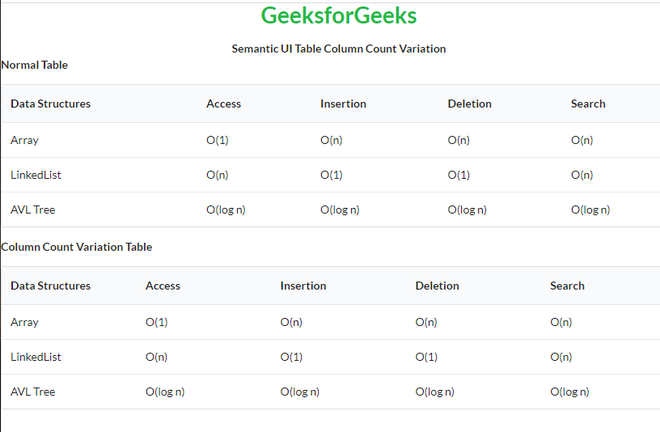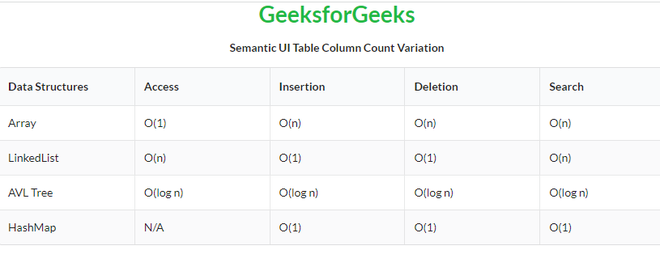Open in App
Not now

# Semantic-UI Table Column Count Variation

• Last Updated : 22 Mar, 2022

Semantic UI is an open-source framework that uses CSS and jQuery to build great user interfaces. It is the same as a bootstrap for use and has great different elements to use to make your website look more amazing. It uses a class to add CSS to the elements. Tables are an easy way to organize a lot of data. A table is an arrangement of data in rows and columns, or possibly in a more complex structure. Tables are widely used in communication, research, and data analysis. Tables are useful for various tasks such as presenting text information and numerical data. It can be used to compare two or more items in the tabular form layout. Tables are used to create databases. An HTML table and a Semantic UI table both are the same structurally. In the case of Column Count Variation, a table specifies its column count to divide its content evenly.

Semantic UI Table Column Count Variation Classes:

• table: This class creates a table in Semantic UI.
• column: This class helps to divide the content evenly based on column count.
• celled: This class divides each row of our table into separate cells.

Syntax:

```<table class="ui number-class column table">
<tr>
<td></td>
...
</tr>
...
</table>```

Example 1: This is a basic example illustrating the Table difference between a normal table and  Column Count Variation Table made using Semantic UI.

## HTML

 ` ` `<``html``> ` ` `  `<``head``> ` `    ``<``title``>Semantic UI Table Column Count Variation ` `    ``<``link` `href``= ` `    ``"https://cdnjs.cloudflare.com/ajax/libs/semantic-ui/2.4.1/semantic.min.css"`  `    ``rel``=``"stylesheet"` `/> ` `    ``<``script` `src``=``"https://code.jquery.com/jquery-3.1.1.min.js"` `        ``integrity``=``"sha256-hVVnYaiADRTO2PzUGmuLJr8BLUSjGIZsDYGmIJLv2b8="`  `        ``crossorigin``=``"anonymous"``> ` `    ``<``script` `src``= ` `    ``"https://cdnjs.cloudflare.com/ajax/libs/semantic-ui/2.4.1/semantic.min.js"``> ` `    `` ` ` ` ` `  `<``body``> ` `    ``<``center``> ` `        ``<``h1` `class``=``"ui green header"``>GeeksforGeeks ` `        ``<``strong``>Semantic UI Table Column Count Variation ` `    `` ` `    ``<``b``>Normal Table ` `    ``<``table` `class``=``"ui table"``> ` `        ``<``thead``> ` `            ``<``tr``> ` `                ``<``th``>Data Structures ` `                ``<``th``>Access ` `                ``<``th``>Insertion ` `                ``<``th``>Deletion ` `                ``<``th``>Search ` `            `` ` `        `` ` `        ``<``tbody``> ` `            ``<``tr``> ` `                ``<``td``>Array ` `                ``<``td``>O(1) ` `                ``<``td``>O(n) ` `                ``<``td``>O(n) ` `                ``<``td``>O(n) ` `            `` ` `            ``<``tr``> ` `                ``<``td``>LinkedList ` `                ``<``td``>O(n) ` `                ``<``td``>O(1) ` `                ``<``td``>O(1) ` `                ``<``td``>O(n) ` `            `` ` `            ``<``tr``> ` `                ``<``td``>AVL Tree ` `                ``<``td``>O(log n) ` `                ``<``td``>O(log n) ` `                ``<``td``>O(log n) ` `                ``<``td``>O(log n) ` `            `` ` `        `` ` `    `` ` `    ``<``b``>Column Count Variation Table ` `    ``<``table` `class``=``"ui five column table"``> ` `        ``<``thead``> ` `            ``<``tr``> ` `                ``<``th``>Data Structures ` `                ``<``th``>Access ` `                ``<``th``>Insertion ` `                ``<``th``>Deletion ` `                ``<``th``>Search ` `            `` ` `        `` ` `        ``<``tbody``> ` `            ``<``tr``> ` `                ``<``td``>Array ` `                ``<``td``>O(1) ` `                ``<``td``>O(n) ` `                ``<``td``>O(n) ` `                ``<``td``>O(n) ` `            `` ` `            ``<``tr``> ` `                ``<``td``>LinkedList ` `                ``<``td``>O(n) ` `                ``<``td``>O(1) ` `                ``<``td``>O(1) ` `                ``<``td``>O(n) ` `            `` ` `            ``<``tr``> ` `                ``<``td``>AVL Tree ` `                ``<``td``>O(log n) ` `                ``<``td``>O(log n) ` `                ``<``td``>O(log n) ` `                ``<``td``>O(log n) ` `            `` ` `        `` ` `    `` ` ` ` ` `  ``

Output:Example 2: This is a basic example illustrating Table Striped Celled Column Count Variation Table made using Semantic UI.

## HTML

 ` ` `<``html``> ` ` `  `<``head``> ` `    ``<``title``>Semantic UI Table Column Count Variation ` `    ``<``link` `href``=``"https://cdnjs.cloudflare.com/ajax/libs/semantic-ui/2.4.1/semantic.min.css"`  `    ``rel``=``"stylesheet"` `/> ` `    ``<``script` `src``=``"https://code.jquery.com/jquery-3.1.1.min.js"` `        ``integrity``=``"sha256-hVVnYaiADRTO2PzUGmuLJr8BLUSjGIZsDYGmIJLv2b8="`  `        ``crossorigin``=``"anonymous"``> ` `    ``<``script` `src``=``"https://cdnjs.cloudflare.com/ajax/libs/semantic-ui/2.4.1/semantic.min.js"``> ` `    `` ` ` ` ` `  `<``body``> ` `    ``<``center``> ` `        ``<``h1` `class``=``"ui green header"``>GeeksforGeeks ` `        ``<``strong``>Semantic UI Table Column Count Variation ` `    `` ` `    ``<``table` `class``=``"ui five column striped table celled"``> ` `        ``<``thead``> ` `            ``<``tr``> ` `                ``<``th``>Data Structures ` `                ``<``th``>Access ` `                ``<``th``>Insertion ` `                ``<``th``>Deletion ` `                ``<``th``>Search ` `            `` ` `        `` ` `        ``<``tbody``> ` `            ``<``tr``> ` `                ``<``td``>Array ` `                ``<``td``>O(1) ` `                ``<``td``>O(n) ` `                ``<``td``>O(n) ` `                ``<``td``>O(n) ` `            `` ` `            ``<``tr``> ` `                ``<``td``>LinkedList ` `                ``<``td``>O(n) ` `                ``<``td``>O(1) ` `                ``<``td``>O(1) ` `                ``<``td``>O(n) ` `            `` ` `            ``<``tr``> ` `                ``<``td``>AVL Tree ` `                ``<``td``>O(log n) ` `                ``<``td``>O(log n) ` `                ``<``td``>O(log n) ` `                ``<``td``>O(log n) ` `            `` ` `            ``<``tr``> ` `                ``<``td``>HashMap ` `                ``<``td``>N/A ` `                ``<``td``>O(1) ` `                ``<``td``>O(1) ` `                ``<``td``>O(1) ` `            `` ` `        `` ` `    `` ` ` ` ` `  ``

Output:My Personal Notes arrow_drop_up
Related Articles